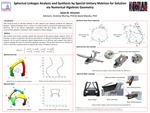## Stander Symposium Projects

#### Presenter(s)

Saleh M Almestiri

#### Description

Numerical algebraic geometry is the field that studies the computation and manipulation of the solution sets of systems of polynomial equations. The goal of this research is to formulate spherical linkages analysis and design problems via a method suited to employ the tools of numerical algebraic geometry. Specifically, equations are developed using special unitary matrices that naturally use complex numbers to express sphysical and joint parameters in a mechanical system. Unknown parameters expressed as complex numbers readily admit solution by the methods of numerical algebraic geometry. This work illustrates their use by analyzing the spherical four-bar and Watt I linkages. I addition, special unitary matrices are utilized to solve the five orientation synthesis of a spherical four-bar linkage. Additionally, synthesis equation were formulated for Watt I linkage. The numerical algebraic geometry software used throughout is Bertini.

4-5-2017

#### Project Designation

Andrew P. Murray, David H. Myszka

Mechanical and Aerospace Engineering

#### Keywords

Stander Symposium projectCOinS